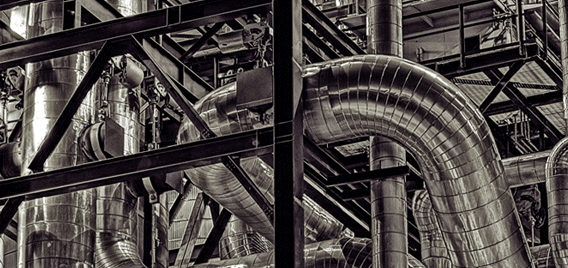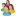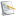### Chemical and Process Engineering Resources## Using Equivalent Lengths of Valves and Fittings

Nov 08 2010 01:20 PM | pleckner in Fluid FlowQuestion #1

Could you please give me in layman terms a better definition for K values. I know that K is defined as "the number of velocity heads lost"...But what exactly does that mean???

Mr. Leckner's reply to this question:

 Well, I'll try to give you the Chemical Engineer's version of the layman answer. Velocity of any fluid increases through pipes, valves and fittings at the expense of pressure. This pressure loss is referred to as head loss. The greater the head loss, the higher the velocity of the fluid. So, saying a velocity head loss is just another way of saying we loose pressure due to and increase in velocity and this pressure loss is measured in terms of feet of head. Now, each component in the system contributes to the amount of pressure loss in different amounts depending upon what it is. Pipes contribute fL/D where L is the pipe length, D is the pipe diameter and f is the friction factor. A fitting or valve contributes K. Each fitting and valve has an associated K.

Â

Question #2

It appears that the K values in CRANE TP-410 were established using a liquid (water) flow loop. Is this K value also valid for compressible media systems? (Can a K value be used for both compressible and incompressible service?)

Mr. Leckner's reply to this question:

 Crane also tested their system on steam and air. Now, this is where things get sticky. As per CRANE TP-410, K values are a function of the size and type of valve or fitting only and is independent of fluid and Reynolds number. So yes, you can use it in ALL services, including two-phase flow.Â  However, as I point out towards the end of my article, there is now evidence that shows using a single K value for the valve and fitting is not correct and that K is indeed a function of both Reynolds number and fitting/valvegeometry. I reference an article by Dr. Ron Darby of Texas A&M University which can be found in Chemical Engineering Magazine, July 1999. Dr. Darby just published a second article on the subject which can be found in Chemical Engineering Magazine, April 2001.I don't believe there is any question as to the proper way to use K values in pressure drop calculations. The only question is whether industry will accept the new data.

Â

Question #3

When answering my first question, you stated:Â  'Velocity of any fluid increases through pipes, valves and fittings at the expense of pressure.'Â  When you say this, you are talking about compressible (gas) flow right?Â  For example, in a pipe of constant area, the velocity of a gas would increase as the fluid traveled down the pipe (due to the decreasing pressure). Â  However, the velocity of a liquid would remain constant as it traveled down the same pipe (even with the decreasing pressure).Â  Is this a correct statement?

Mr. Leckner's reply to this question:

 Sorry for the confusion. Yes to both of your questions. If you look at the Bernoulli equation, you will see that velocity cancels out for a liquid as long as there is no change in pipe size along the way and pressure drop is only a function of frictional losses and a change in elevation.However, the K value of a fitting is still a quantifier of the head loss (frictional loss) in that fitting and this head loss is still calculated as the velocity head of the liquid (V^2/2g). So in essence, you still achieve a liquid velocity at the expense of pressure loss; the velocity head just happens to be constant. Read section 2-8 in CRANE TP-410. They define the velocity head as a decrease in static head due to velocity.The big thing is not to get too hung up on the definitions and just remember you can't have flow unless you have a driving force and that force is differential pressure. Also, in a piping system there is frictional losses which comes from the pipe and all fittings and valves. The use of K is just a way of quantifying the frictional component of the fittings and valves. You can even put the piping friction in terms of K by using fL/D for the pipe and multiplying that by V^2/2g.I hope this helps. If you are still confused, let me know and I'll just explain it again but I'll try to do it in a different way. Sometimes, a concept just needs to be re-worded and I'm willing to spend as much time on this as you need.

Â

Question #4

I'm reading the Crane Technical Paper #410 and I have the following

Page 2-8 of TP 410 states that:
"Velocity in a pipe is obtained at the expense of static head".Â  This makes sense and Equation 2-1 shows this relationship where the static head is converted to velocity head.Â  However, there is no diameter associated with this.Â  So is it correct to say based on equation 2-1 that if you had a barrel of water with a short length of pipe attached to the bottom that discharged to atmosphere, and in this barrel you had 5 feet of water (5' of static head), the resulting water velocity would be 17.94 ft/sec (regardless
of the pipe diameter).

Maybe the real question is how do you use equation 2-1.Â  Do you have to know the velocity and then you can calculate the headloss?Â  And why does equation 2-1 and equation 2-3 seem to show headloss equaling two different things?

Also, why does it say that a diameter is always associtated with the K value, when as I mentioned above there is no diameter associated with equation 2-1?

Maybe I'm trying to read into all of this too deeply, but I still do not feel that I fully grasp what page 2-8 is trying to reveal.

Mr. Leckner's reply to this question:

 You need a diameter to get velocity. Velocity is lenght/time (for example, feet/sec). Flow is usually given in either mass units (weight/time or lb/hr for example) or in volumetric units (cubic feet per minute for example). To get velocity, you need to divide the volumetric flow by a cross sectional area (square feet). To get an area, you need a diameter. So the velocity is always based on some diameter.As I show in my paper, equation 2-1 is just the basis of the velocity head. To get the frictional loss, you need to know the contribution of each component in the system; pipe, fitting and valve. To get that contribution, you use 'K' (equation 2-2). Each component has an associated 'K' value. You multiply the velocity head by the appropriate 'K' value. Equation 2-3 is just another way of expressing the same thing. As you can see, this means you can calculate a 'K' for a component such as a pipe using the formula fL/D as shown in Equation 2-3. Again, I explain this in my paper so I would suggest you re-read it.I would also suggest you look at the examples in CRANE. There are many of them in Chapter 4.'K' is associated with the velocity and therefore the diameter. Look at the values for 'K' in CRANE (starting on page A-26). You will see that for the most part, K is a function of a constant times the friction factor at fully turbulent flow. This friction factor changes with pipe diameter as shown on page A-26. Again, re-read my paper and look at the examples in Chapter 4.

### Fluid Flow Articleslidachang

yeal,thank you very much,i got a lot from  this topic.ksb
In Crane Tech Paper 410 (1982 version), Example 4-14 shows a 5"x4" Reducing Elbow which they model as a straight size 5" elbow and a sudden enlargement 4" to 5". In step 4 of Example 4-14, they calculate a pressure increase (negative pressure drop) according to the Bernoulli theorem, because the water slows down as it flows from the smaller diameter to the larger diameter. I was wondering why you didn't include the velocity change in your Sulfuric Acid example since it flows from the 1" pump discharge nozzle through the reducer to the 3" pipe. Since the velocity in the 1" nozzle is 31.22 ft/s, the Bernoulli dP would be -11.72 psi plus the frictional dP of 7.24 psi for a total pressure drop of -4.48 psi which is a pressure increase.I'm a bit confused by the preferred equations to be used. The only equation that produces the 11.73 psi dP for the system described is Equation 2, yet Equations 6 & 7 seem to be preferred.

•Industrial Forum

•Student Forum

•Refining Forum

•Simulation Forum

•Relief Devices Forum

•Tank Venting Forum

•Separation Technology

•Calculations and Tips

•Fluid Flow

•Heat Transfer

•Maintenance and Repair

•Utilities

•Safety and Pressure Relief

•Bulk Solids

•Process and Reactions

•Energy

•Other Topics

•For Students

•Physical Properties

•Archived Articles